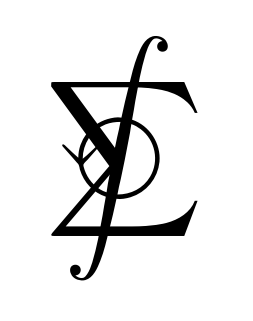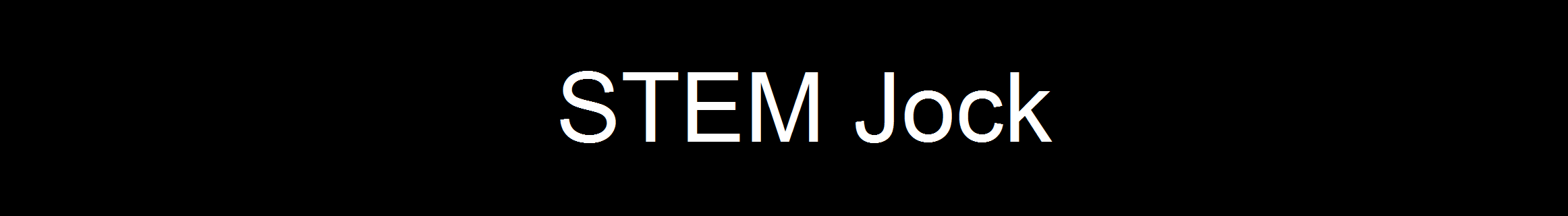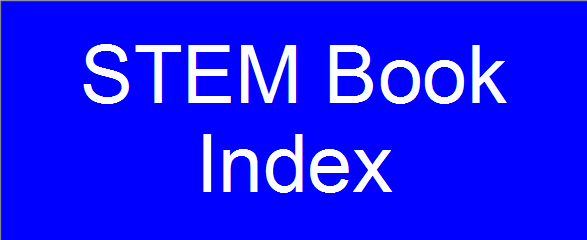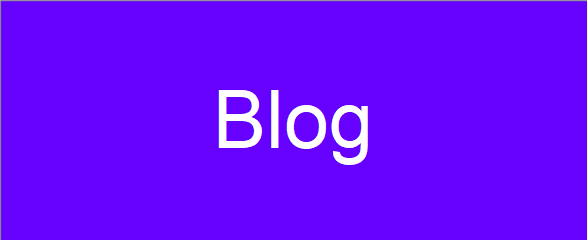On this webpage you will find my solutions to the problems on projecteuler.net. All code shall be written in C. You can edit and compile C programs for free here. If you find my work useful, please consider making a donation. Thank you.

 Problem 1: Multiples of 3 and 5 Problem 6: Sum square difference Problem 2: Even Fibonacci numbers Problem 7 | Code: 10001st prime Problem 3 | Code: Largest prime factor Problem 8: Largest product in a series Problem 4 | Code: Largest palindrome product Problem 9 | Code: Special Pythagorean triplet Problem 5 | Code: Smallest multiple Problem 10 | Code: Summation of primes
 Problem 11: Largest product in a grid Problem 16: Power digit sum Problem 12 | Code: Highly divisible triangular number Problem 17: Number letter counts Problem 13 | Code: Large sum Problem 18: Maximum path sum I Problem 14 | Code: Longest Collatz sequence Problem 19: Counting Sundays Problem 15: Lattice paths Problem 20: Factorial digit sum